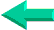Special

Excel Functions

2003-2010

## Lesson 19: VLOOKUP function in Excel

The VLOOKUP function in Excel searches for a value in the leftmost column of a table and returns the value on the same row of another column to the right. So VLOOKUP looks up for " John" in column A and his address, his age or his balance appear in either columns B, C or D.

Here are the most common questions from users:

1- What if the lookup column in  my table is not the leftmost column?

With VLOOKUP function it is complicated. The table needs to be reorganized to make the lookup column the left most column of the table. With INDEX/MATCH the lookup column can be anywhere.

2- What if the values in my lookup column are not in alphabetical order?

With INDEX/MATCH instead of VLOOKUP it doesn't matter. With VLOOKUP you need to add a 5th  argument to your formula (FALSE). This means that each time a new row is added   the table MUST be re-sorted.

3- Can 2 criteria be used with VLOOKUP? For example, can I find John (A) Baxter (B)'s address ( C) or can I find the price (D)  of a 1978 (A), Chevrolet ( B), Malibu (C).

Yes, but it is complicated. Create a new column in which the values of the criteria columns are concatenated and use this new column as criteria (first and last name or year, make and model). Remember that this new column must be the leftmost column of the table.

There is no need to create a new column with SUMPRODUCT or INDEX/MATCH in array format.

4- In a sales database can I sum all the sales (E) of one product (B) with VLOOKUP?

No, use SUMPRODUCT. For example, if in A you have dates, in B store numbers,   in C products and in D amounts,   you can with SUMPRODUCT sum the sales of a product, in a certain store and on a certain date.

VLOOKUP is a function inherited from Lotus-123. There is a more powerful and less limited way in Excel. It is the INDEX/MATCH formulas. INDEX/MATCH can replace all lookup functions (VLOOKUP, HLOOKUP and LOOKUP).

### The VLOOKUP function in Excel

This function searches for values in the left-most column of a table and returns the values on the same row of another column to the right. So VLOOKUP looks up for "John" in column A and get his address, his age or his balance that are in columns B, C or D.

VLOOKUP in Excel is used in two situations:

- On an invoice or other documents you enter the name of a client and in the next few cells an Excel VLOOKUP formula brings in the address 1, address 2, the city, State and ZIP Code. Less manual entries equals less mistakes. On the same invoice you type in the product number and in other cells its description, unit and unit price are carried over by Excel VLOOKUP's.

- You have a set of sales data and you want to subtotal   sales by cities. Unfortunately the city in which the client lives in not part of the set of data. This information exists in another set of data that you import on sheet 2. With Excel VLOOKUP you can bring the name of the city within your first set of data on sales and calculate  the subtotals by city.

IMPORTANT NOTE: The values that your formula is looking up through MUST BE IN ASCENDING ORDER (a, b, c, d) unless the fifth argument is set to FALSE.

Here is a basic formula: =VLOOKUP("John" ,A1:G32,2, FALSE)

The syntax for these formulas is  as follows the first argument ("John" ) is what VLOOKUP is looking for, the second argument (A1:G32) means to search a value in the table A1 to G32 looking up for " John" in column A, the third argument (2) is the column from which the answer is extracted, and the fifth argument (FALSE) tells Excel that you are looking for an EXACT  match and not the next lower value. If the fifth argument is omitted or set to TRUE the formula will return the answer for the next lower value if it doesn't find EXACTLY what you are looking for and if the values are sorted in ascending order. For example if looking up for John and the formula only finds Albert and Susan it will return the answer for Albert.
=VLOOKUP("John" ,A1:G32,2, FALSE) looks for " John" in the first column (A) of the range (A1:G32) and returns the value of the cell in the 2nd column (B) same row.

Use   the address of a cell in which the value as first argument in the formula (ex: J20) is changed
=VLOOKUP(J20,A1:G32,2, FALSE) looks for whatever value is in cell J20 in the first column (A) of the range (A1:G32) and returns the value of the cell in the 2nd column (B) same row.

Remember to ALWAYS use " FALSE" as fifth argument and to make sure that the values in the column that VLOOKUP is looking up within are in ascending order.

VLOOKUP is always looking up within the first column of the table that is submitted as second argument. If column C is to be the look up column change the second argument to C1:G32.
=VLOOKUP(J20,C1:G32,2, FALSE).Back home